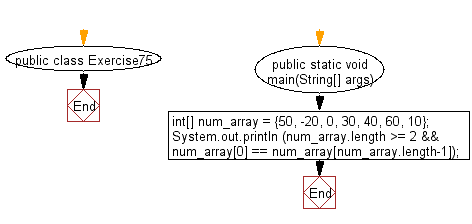﻿ Java: Test if the first and last value of an array are same# Java Exercises: Test if the first and the last element of an array of integers are same

## Java Basic: Exercise-75 with Solution

Write a Java program to test if the first and the last element of an array of integers are same. The length of the array must be greater than or equal to 2.

Sample Solution:

Java Code:

``````import java.lang.*;
public class Exercise75 {
public static void main(String[] args)
{
int[] num_array = {50, -20, 0, 30, 40, 60, 10};

System.out.println (num_array.length >= 2 && num_array ==  num_array[num_array.length-1]);
}
}
```
```

Sample Output:

```false
```

Pictorial Presentation:Flowchart:Java Code Editor:

What is the difficulty level of this exercise?

Test your Programming skills with w3resource's quiz.

﻿

## Java: Tips of the Day

countOccurrences

Counts the occurrences of a value in an array.

Use Arrays.stream().filter().count() to count total number of values that equals the specified value.

```public static long countOccurrences(int[] numbers, int value) {
return Arrays.stream(numbers)
.filter(number -> number == value)
.count();
}
```

Ref: https://bit.ly/3kCAgLb